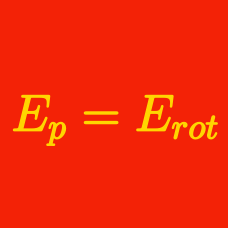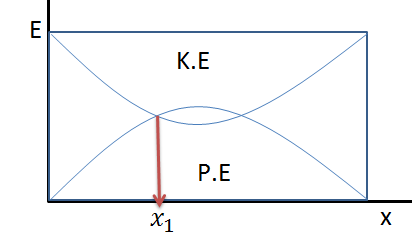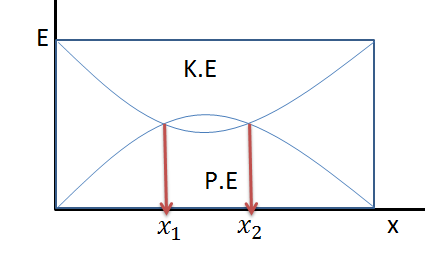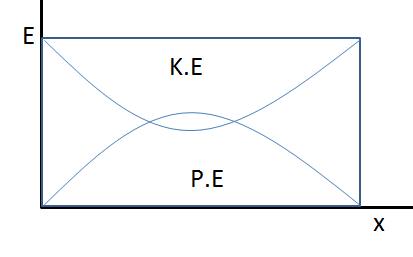Classical Mechanics

# Energy graphsThe figure above shows the kinetic and potential energies of a $6 \text{ kg}$ projectile. At time $t=1\text{ s},$ the kinetic and potential energies of the projectile are equal, and the projectile's horizontal position is $x_1=4\text{ m}.$ If the projectile's initial vertical velocity can be expressed as $\left(a \pm \sqrt{b}\right) \text{ m/s},$ where $a$ and $b$ are positive integers, what is the value of $a+b?$

The air resistance is negligible and the gravitational acceleration is $g= 10 \text{ m/s}^2.$The figure above shows the kinetic and potential energies of a $3 \text{ kg}$ projectile. At times $t=1 \text{ s}$ and $t = t_2,$ the kinetic and potential energies of the projectile are equal, and the projectile's respective horizontal positions at those moments are $x_1=6\text{ m}$ and $x_2 \text{ m}.$ If the horizontal position $x_2$ can be expressed as $x_2= \left(a \pm \frac{6}{5}\sqrt{b}\right) \text{ m },$ where $a$ and $b$ are positive integers, what is the value of $a+b?$

The air resistance is negligible and the gravitational acceleration is $g= 10 \text{ m/s}^2.$The graph above shows the potential and kinetic energies of a $6 \text{ kg}$ projectile that is fired with an initial velocity of $4 \text{ m/s}$ in a direction that makes a $60^\circ$ angle with the horizon. When the projectile's gravitational potential energy reaches its maximum value, what is the speed of the projectile?

Air resistance is negligible and the gravitational acceleration is $g= 10 \text{ m/s}^2.$The figure above shows the kinetic and potential energies of a $8 \text{ kg}$ projectile. At times $t=1 \text{ s}$ and $t = t_2,$ the kinetic and potential energies of the projectile are equal, and the projectile's respective horizontal positions at those moments are $x_1=2\text{ m}$ and $x_2.$ If time $t_2$ can be expressed as $t_2= \left(a \pm \frac{1}{5}\sqrt{b}\right) \text{ s},$ where $a$ and $b$ are positive integers, what is the value of $a+b?$

The air resistance is negligible and the gravitational acceleration is $g= 10 \text{ m/s}^2.$

A $8 \text{ kg}$ ball is fired with an initial velocity of $2 \text{ m/s}$ in a direction that makes a $30^\circ$ angle with the horizon. When the ball reaches its highest point, what is the difference between the ball's kinetic energy and gravitational potential energy?

Air resistance is negligible and the gravitational acceleration is $g= 10 \text{ m/s}^2.$

×